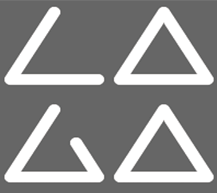# Le LAGAThe Laboratoire Analyse, Géométrie et Applications is a laboratory assiociated with the CNRS (UMR 7539). It is connected to the Galileo Institute, component of the Sorbonne Paris Nord University, and to the Paris 8 university. The LAGA is also associated to several LaBeX : SMP, INFLAMEX and MME-DII. It is comprised of approximatively 90 researchers and teachers-researchers (of which about ten from the CNRS), 7 ITA and BIATSS staff, more than 50 PhD students and welcomes each year more than thirty foreign visitors and post graduate students. The main research topics currently pursued in the laboratory are as follows: arithmetic, algebraic geometry, theory of numbers, category theory, algebraic topology, homotopy theory, representation theory, dynamic systems, ergodic theory, harmonic analysis, linear and nonlinear partial differential equations, microlocal analysis, mathematical physics, spectral theory, numerical analysis, probability and statistics, stochastic analysis, coding and cryptography, image processing.

The laboratory, led by Julien Barral, is structured in seven research teams:

• Arithmetic, algebraic geometry and Applications (AGAA)
• Modelling and scientific computing (MCS)
• Mathematical physics and partial differential equations (PM)
• Mathematics for Biology and Imaging (MBI)
• Probabilities et Statistics (PS).
• Ergodic theory et dynamic systems (SD)
• Algebraic topology (TA)

The laboratory’s scientific activity is embodied by permanent or episodic seminars, as well as the existence of the Mathematics Library. Finally, the laboratory has a vocation to offer a solid frame of work to PhD students coming from the Paris Nord Sorbonne University’s mathematics department’s master degrees, but also to students from other mathematics master degrees of the Parisian region, the rest of the country and possibly similar courses abroad.

LAGA’s website

Scroll to Top